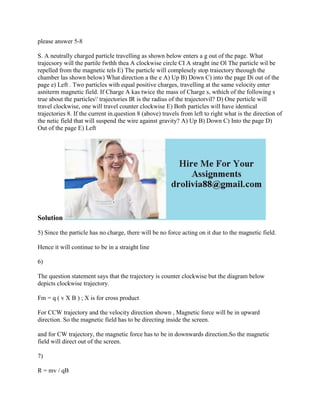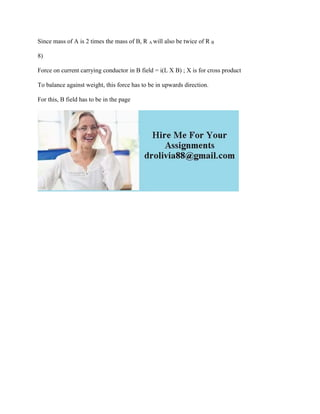Diese Präsentation wurde erfolgreich gemeldet.

# please answer 5-8 S- A neutrally charged particle travelling as shown.docx

Anzeige
Anzeige
Anzeige
Anzeige
Anzeige
Anzeige
Anzeige
Anzeige
Anzeige
Anzeige
Anzeige×

1 von 2 Anzeige

# please answer 5-8 S- A neutrally charged particle travelling as shown.docx

S. A neutrally charged particle travelling as shown below enters a g out of the page. What trajecsory will the partile fwthh thea A clockwise circle CI A straght ine Ol The particle wil be repelled from the magnetic tels E) The particle will complesely stop traiectory theough the chamber las shown below) What direction a the e A) Up B) Down C) into the page Di out of the page e) Left . Two particles with equal positive charges, travelling at the same velocity enter asniterm magnetic field. If Charge A kas twice the mass of Charge s, wthich of the following s true about the particles\' trajectories IR is the radius of the trajectorvil? D) One perticle will travel clockwise, one will travel counter clockwise E) Both particles will have identical trajectories 8. If the current in.question 8 (above) travels from left to right what is the direction of the netic field that will suspend the wire against gravity? A) Up B) Down C) Into the page D) Out of the page E) Left
Solution
5) Since the particle has no charge, there will be no force acting on it due to the magnetic field.
Hence it will continue to be in a straight line
6)
The question statement says that the trajectory is counter clockwise but the diagram below depicts clockwise trajectory.
Fm = q ( v X B ) ; X is for cross product
For CCW trajectory and the velocity direction shown , Magnetic force will be in upward direction. So the magnetic field has to be directing inside the screen.
and for CW trajectory, the magnetic force has to be in downwards direction.So the magnetic field will direct out of the screen.
7)
R = mv / qB
Since mass of A is 2 times the mass of B, R A will also be twice of R B
8)
Force on current carrying conductor in B field = i(L X B) ; X is for cross product
To balance against weight, this force has to be in upwards direction.
For this, B field has to be in the page
.

S. A neutrally charged particle travelling as shown below enters a g out of the page. What trajecsory will the partile fwthh thea A clockwise circle CI A straght ine Ol The particle wil be repelled from the magnetic tels E) The particle will complesely stop traiectory theough the chamber las shown below) What direction a the e A) Up B) Down C) into the page Di out of the page e) Left . Two particles with equal positive charges, travelling at the same velocity enter asniterm magnetic field. If Charge A kas twice the mass of Charge s, wthich of the following s true about the particles\' trajectories IR is the radius of the trajectorvil? D) One perticle will travel clockwise, one will travel counter clockwise E) Both particles will have identical trajectories 8. If the current in.question 8 (above) travels from left to right what is the direction of the netic field that will suspend the wire against gravity? A) Up B) Down C) Into the page D) Out of the page E) Left
Solution
5) Since the particle has no charge, there will be no force acting on it due to the magnetic field.
Hence it will continue to be in a straight line
6)
The question statement says that the trajectory is counter clockwise but the diagram below depicts clockwise trajectory.
Fm = q ( v X B ) ; X is for cross product
For CCW trajectory and the velocity direction shown , Magnetic force will be in upward direction. So the magnetic field has to be directing inside the screen.
and for CW trajectory, the magnetic force has to be in downwards direction.So the magnetic field will direct out of the screen.
7)
R = mv / qB
Since mass of A is 2 times the mass of B, R A will also be twice of R B
8)
Force on current carrying conductor in B field = i(L X B) ; X is for cross product
To balance against weight, this force has to be in upwards direction.
For this, B field has to be in the page
.

Anzeige
Anzeige

### please answer 5-8 S- A neutrally charged particle travelling as shown.docx

1. 1. please answer 5-8 S. A neutrally charged particle travelling as shown below enters a g out of the page. What trajecsory will the partile fwthh thea A clockwise circle CI A straght ine Ol The particle wil be repelled from the magnetic tels E) The particle will complesely stop traiectory theough the chamber las shown below) What direction a the e A) Up B) Down C) into the page Di out of the page e) Left . Two particles with equal positive charges, travelling at the same velocity enter asniterm magnetic field. If Charge A kas twice the mass of Charge s, wthich of the following s true about the particles' trajectories IR is the radius of the trajectorvil? D) One perticle will travel clockwise, one will travel counter clockwise E) Both particles will have identical trajectories 8. If the current in.question 8 (above) travels from left to right what is the direction of the netic field that will suspend the wire against gravity? A) Up B) Down C) Into the page D) Out of the page E) Left Solution 5) Since the particle has no charge, there will be no force acting on it due to the magnetic field. Hence it will continue to be in a straight line 6) The question statement says that the trajectory is counter clockwise but the diagram below depicts clockwise trajectory. Fm = q ( v X B ) ; X is for cross product For CCW trajectory and the velocity direction shown , Magnetic force will be in upward direction. So the magnetic field has to be directing inside the screen. and for CW trajectory, the magnetic force has to be in downwards direction.So the magnetic field will direct out of the screen. 7) R = mv / qB
2. 2. Since mass of A is 2 times the mass of B, R A will also be twice of R B 8) Force on current carrying conductor in B field = i(L X B) ; X is for cross product To balance against weight, this force has to be in upwards direction. For this, B field has to be in the page